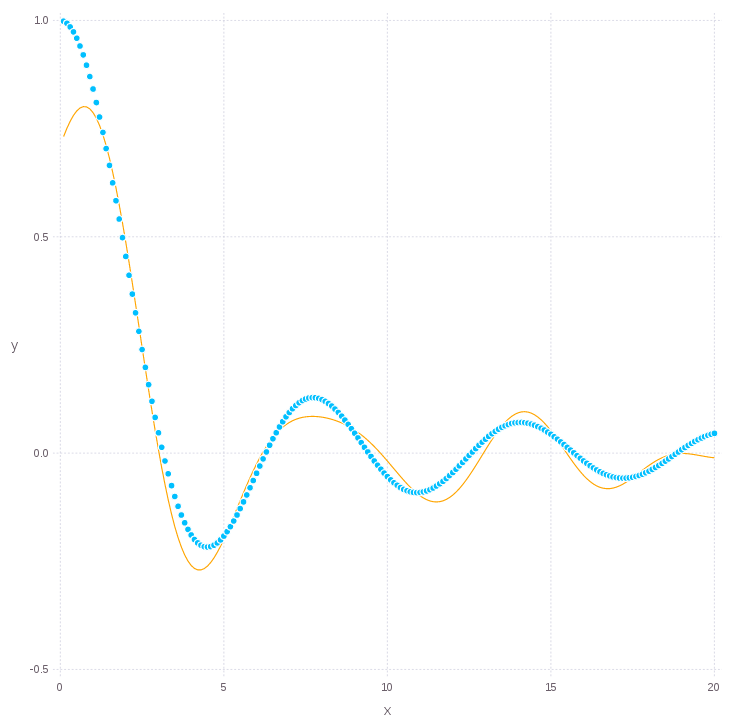# Examples & notebooks¶

## Prerequisites¶

Please refer to Julia downloads page for installing Julia language and all dependencies. The instructions for installing the SALSA package can be found here. Some additional plotting and data management packages might be required to run examples below (like `Gadfly`, `MAT` or `DataFrames`). If you prefer Python-style notebooks please refer to the Project Jupyter and IJulia package for instructions. In this section we provide code snippets which can be easily copied into the Julia console or Jupyter notebook. Please find an explanation on examples and functional `IJulia` notebooks online.

This example provides a use-case for nonlinear classification using Nyström approximation and Area Under ROC Curve (with 100 thresholds) as a cross-validation criterion.

```using SALSA, MAT

ripley = matread(joinpath(Pkg.dir("SALSA"), "data", "ripley.mat")); srand(123);
model = SALSAModel(NONLINEAR, PEGASOS(), LOGISTIC, validation_criterion=AUC(100));
model = salsa(ripley["X"], ripley["Y"], model, ripley["Xt"]);

range1 = linspace(-1.5,1.5,200);
range2 = linspace(-0.5,1.5,200);
grid = [[i j] for i in range1, j in range2];

Xgrid = foldl(vcat, grid);
Xtest = ripley["Xt"];

yhat = model.output.Ytest;
yplot = map_predict_latent(model,Xgrid);
yplot = yplot - minimum(yplot);
yplot = 2*(yplot ./ maximum(yplot)) - 1;

using DataFrames
df = DataFrame();
df[:X] = Xgrid[:,1][:];
df[:Y] = Xgrid[:,2][:];
df[:class] = yplot[:];

set_default_plot_size(20cm, 20cm);
plot(layer(x=Xtest[yhat.>0,1], y=Xtest[yhat.>0,2], Geom.point, Theme(default_color=colorant"orange")),
layer(x=Xtest[yhat.<0,1], y=Xtest[yhat.<0,2], Geom.point, Theme(default_color=colorant"black")),
layer(df, x="X", y="Y", color="class", Geom.rectbin))
```This example provides a use-case for regression using Nyström approximation and `mse()` (Mean Squared Error) as a criterion in the Leave-One-Out cross-validation defined in MLBase.jl package.

```using SALSA, MLBase

sinc(x) = sin(x)./x;
X = linspace(0.1,20,100)'';
Xtest = linspace(0.1,20,200)'';
Y = sinc(X);
srand(1234);

model = SALSAModel(NONLINEAR, PEGASOS(), LEAST_SQUARES,
cv_gen=Nullable{CrossValGenerator}(LOOCV(100)),
validation_criterion=MSE(), process_labels=false, subset_size=5.0);
model = salsa(X, Y, model, Xtest);